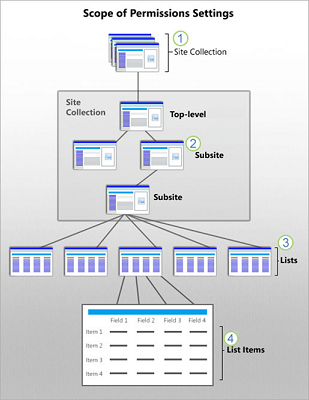# SharePoint 中的各个权限级别

## 权限继承概述• 网站集管理员为整个集合配置首要网站或根网站的权限。

• 如果你是网站所有者，可以更改网站的权限设置，从而停止网站的权限继承。

• 列表和库会从其所属的网站继承权限。如果你是网站所有者，则可以停止权限继承，并更改列表或库的权限设置。

• 列表项和库文件从其父列表或库继承权限。如果拥有列表或库的控制权限，则可以停止权限继承，并直接在特定项目中更改权限设置。

用户可以通过与不具有访问权限的人员共享文档或项来中断列表或库项的默认权限继承，知道这一点非常重要。在这种情况下，SharePoint 会自动停止文档的继承。

## 默认权限级别

Microsoft 365 订阅创建一个名为“除外部用户外的所有人”的安全组，该组包含添加到 Microsoft 365 目录中的每个人（明确添加为外部用户的人除外）。 此安全组会自动添加到新式团队网站上的“成员”组，并具有“公开”隐私设置，好让 Microsoft 365 中的用户可以访问并编辑 SharePoint 网站。 另外，对于创建为“私人”的新式团队网站，“除外部用户之外的所有人”都不能被授予任何权限，并且必须明确地授予人员权限。 此外，Microsoft 365 订阅会创建一个名为"公司管理员"的安全组，该组包含 Microsoft 365 管理员（如全局管理员和帐务管理员）。 此安全组已添加到网站集管理员组。 有关详细信息，请参阅默认 SharePoint 组

## 权限级别和 SharePoint 组• 您为网站选择的模板

• SharePoint 管理员是否在网站上创建了具有特定用途（如搜索）的唯一权限集

X
X

X
X

X
X

X
X

X
X
X

X
X

X
X

x

X
X
X
X
X
X

X
X
X
X
X
X
X
X

X
X
X
X
X
X
X
X
X

X
X

X
X
X
X
X
X
X
X
X

X
X

X
X
X
X
X
X
X
X

X
X
X
X
X
X
X
X
X

X
X
X
X
X
X
X
X
X
X

X
X
X
X
X
X

X
X
X
X

X
X
X
X

X
X
X
X
X
X

X
X
X
X
X
X

X
X
X
X
X
X

X
X
X
X
X
X
X
X
X

X
X
X

X
X
X
X
X
X
X
X

X
X
X
X
X
X
X
X

X
X
X
X
X
X

X
X
X
X
X
X
X
X

X
X
X
X
X
X
X
X

X
X
X
X
X
X

X
X
X
X
X
X

X
X
X
X
X
X

## 权限和依赖权限

SharePoint 权限可以依赖于其他 SharePoint 权限。例如，要查看某个项目，必须能够将其打开。这样，"查看项目"权限就依赖于"打开"权限。

X

X
X

X

X
X

X
X